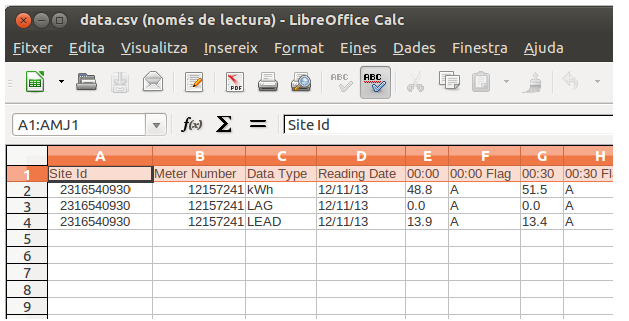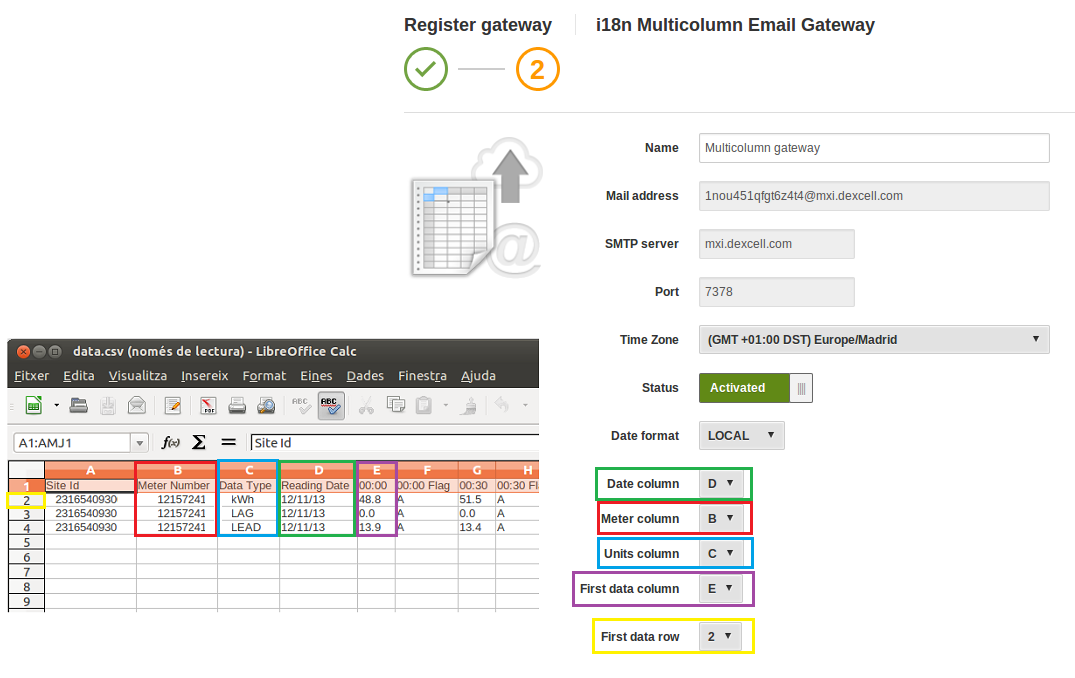# Multicolumn CSV gateway

### Multicolumn CSV gateway

In this article we will learn how to register a "Email Multicolumn CSV" gateway useful to send daily data through email to DEXCell Energy Manager.

1. First of all, we go to Settings > Gateways > Register new Gateway > and we select "Email Multicolumn CSV"
2. Then, we have to indicate the basic information corresponding to the file we are going to send periodically to DEXCell Energy Manager.
3. Here, we will obtain the email address we need to send the data.
4. Finally, we have to indicate which column corresponds to the date, the meter, the units and the first data column.

### Example

Now, we are going to show a real example in order to understand how to register the gateway. In this case, we have the following example obtained from IMServ (for more information, click here).Now, we have to indicate the basic configuration on DEXCell Energy Manager and relate it with the excel file.So, in our example, we have:
- The "date" is indicated on the de column D
- The "meter ID number" is on the column B
- The "units" are on the columns C
- The "first data" column is the E
- The first data row in our excel file is the 2th.

- kWh: Active Energy (parameter 402)
- LAG: Reactive Energy (parameter 404)
- LEAD: Capacitive Reactive Energy (parameter 408)

### Sending data to different energy sources

If in your project you need to send different energy sources (electricity, gas, water, etc,) then in the "Column C" you need to specify which parameter are you sending to DEXCell Energy Manager according to the following list.

For example, if you would like to introduce Gas Energy, then you need to edit your file and specify the parameter "420" on it. If you want to introduce Water Volume, then the parameter 901 is the one to use.

Please note that this gateway will accumulate the values by default. This causes the impossibility of inserting data of non-continuous variables, such as power. If you want to insert discrete variables, we would recommend to you to whether manipulate the variable's values so it becomes continuous (inserting energy instead of power through hourly averages for instance) or use a different gateway.